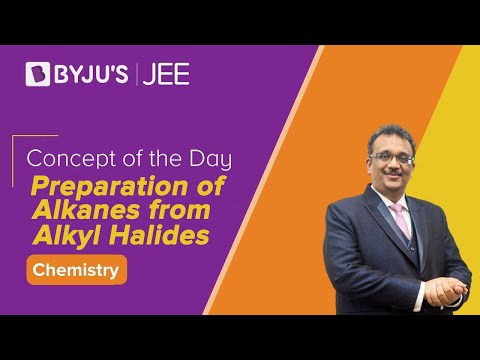Checkout JEE MAINS 2022 Question Paper Analysis : Checkout JEE MAINS 2022 Question Paper Analysis :

# Alkanes Questions

Alkanes are saturated hydrocarbons with a general formula CnH2n+2. Based on their structure, they are further categorized into linear straight chain alkanes, branched chain alkanes and cycloalkanes. They are odorless, colorless and are insoluble in water. The melting point and boiling point of alkanes increases with increase in molecular weight. The chemical reactivity of alkanes is less as they are highly stable compounds. Alkanes are industrially important as fuels.

 Definition: The alkanes with C1 to C4 carbon atoms are gasses, C5 to C17 are liquids and alkanes with carbon atoms greater than 18 are solids at room temperature. Methane (CH4), Ethane (C2H6), Propane (C3H8) are the first three alkanes.

## Alkane Chemistry Questions with Solutions

Q1. What kind of reactions do alkanes undergo?

Answer: The two most important reactions of alkanes are combustion and halogenation.

Combustion: Alkanes undergo a combustion reaction to give carbon dioxide and water.

Halogenation: Alkanes undergo halogenation reaction to give haloalkane.

Q2. Give an example each of combustion reaction and halogenation reaction of alkanes

Answer: Combustion reaction: Methane undergoes combustion reaction to give carbon dioxide and water.

CH4 + 2O2 → CO2 + 2H2O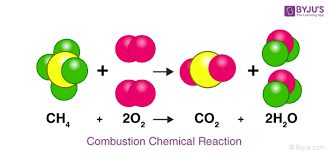Halogenation reaction: Ethane undergoes bromination reaction to give ethyl bromide in the presence of heat or light.

$$\begin{array}{l}C_{2}H_{6} + Br_{2} \overset{\Delta }{\rightarrow} C_{2}H_{5}Br + HBr\end{array}$$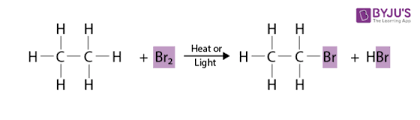Q3. Choose the alkane from the following

(a) Hexane

(b) Hexene

(c) Hexyne

(d) Hexanol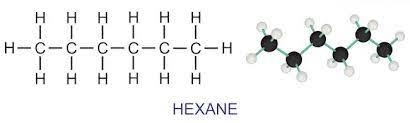Q4. Choose the correct molecular formula of alkane from the following

(a) C4H10

(b) C5H10

(c) C6H6

(d) C4H8

C4H10 is the alkane butane.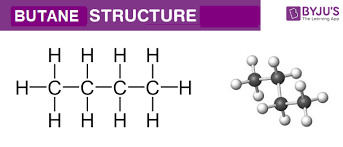Q5. Why are alkanes called saturated hydrocarbons?

Answer: Alkanes are called saturated hydrocarbons because they do not contain multiple bonds like double bond or triple bond in their structure. The carbon and hydrogen atoms in alkanes are bonded by single covalent bonds.

Q6. What is meant by incomplete combustion?

Answer: Incomplete combustion is a combustion reaction in which poisonous carbon monoxide gas is released. The energy released in incomplete combustion is less than that of complete combustion.

Q7. Match the following items of column 1 with column 2 and choose the correct answer:

Column 1

Column 2

1) C5H12

a) Cyclic alkane

2) C3H6

b) Branched alkane

3)C8H18

c) Linear alkane

Column 1

Column 2

1) C5H12

c) Linear alkane

2) C3H6

a) Cyclic alkane

3)C8H18

b) Branched alkane

Q8. What is the bond angle of alkanes?

Answer: The bond angle of alkanes is 109.5o.

Q9. Arrange n-pentane, iso-pentane and neopentane in decreasing order of their boiling points.

Answer: Boiling point decreases with increase in branching of alkanes. Thus, n-pentane > iso-pentane > neopentane is the decreasing order of boiling points.

Q10. Which metal is used in the preparation of grignard reagent from haloalkanes?

Answer: Magnesium metal is used in the preparation of grignard reagent from haloalkanes.

Q11. Give one use of ethylene dibromide.

Answer: Ethylene dibromide is used as an anti-knocking agent in the combustion chambers to prevent the deposition of lead metal.

Q12. Explain Wurtz reaction.

Answer: The reaction in which 2 moles of alkyl halide react with sodium in presence of dry ether to give alkane is called Wurtz reaction.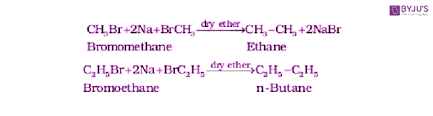Q13. Which compound is most volatile among the following?

(a) Isobutane

(b) n-pentane

(c) 2,2 dimethylpropane

(d) propane

Q14. What is freon-12?

Answer: Dichlorodifluoromethane is known as freon-12. It is a colorless gas and is used as a refrigerant and also as an aerosol spray propellant.

Q15. What is the geometry of a methane molecule?

Answer: Methane molecule is tetrahedral in geometry.

## Practice Questions on Alkanes

Q1.Which among the following has the highest boiling point?

(a) Pentane

(b) Hexane

(c) Heptane

(d) Octane

Q2.What are alkanes also known as?

Q3.What is the product obtained when aluminum carbide is treated with water?

Q4. What is paraffin wax?

(a) Saturated hydrocarbon

(b) Unsaturated hydrocarbon

(c) Alcohol

(d) Carboxylic acid

Q5.What does petroleum mainly consist of?

(a) Aromatic hydrocarbons

(b) Aliphatic hydrocarbons

(c) Aliphatic alcohols

(d) Aliphatic acids

Click the PDF to check the answers for Practice Questions.

## Preparation of Alkanes Explanation